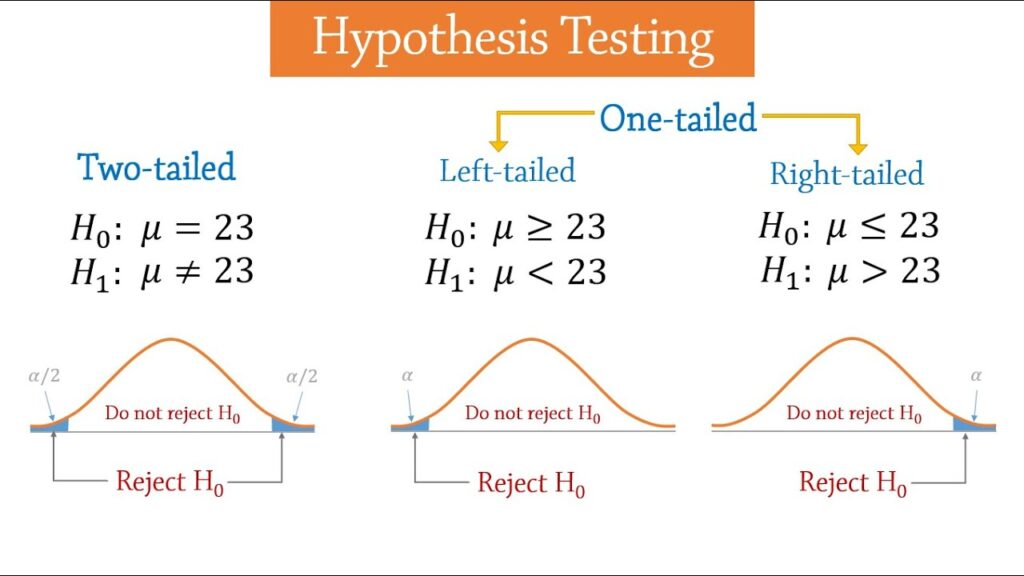# Hypothesis Testing: Upper, Lower, and Two Tailed TestsHypothesis testing is a statistical procedure that helps to evaluate the two mutually exclusive statements about the population. It helps to know which of the statement is best according to the sample data. Test of hypothesis is based on the information and investigator belief about the parameters of population. The testing process involves two competing hypothesis, first one is null hypothesis and the second one is alternative hypothesis.

In the testing procedure critical values indicates the acceptance and rejection region to make the decisions. For convenience, you can give an account to the critical values calculator that allows you to determine the critical values of any tail.Page Contents

## Procedure of Hypothesis:

The procedure of hypothesis testing is based on the ideas; firstly, we need to state the hypothesis as null or alternative. Select the random samples on the base of population of interest and summary statistics. After that we can determine whether the sample data is supportive for null or alternative hypothesis or not. The procedure of testing is summarized into simple steps and they’re:

## Step # 1: Defining the Hypothesis:

In the first step of statistical hypothesis testing, setup the hypothesis and choose the significance level Alpha, which is denoted by α. The significance level is an important factor in statistical hypothesis test. If you know the level of confidence, then simply give a try to the left and right critical value calculator to calculate the critical values of different distributions.

### Step # 2: Test Selection:

Selecting the appropriate test statistic for the distribution is very important step of testing. The test statistic is said to be a single number, which is used to summarize the sampling information.  Z-statistic is the example of the test statistic, it is calculated by using the standard formula of z-test:

If the size of the sample is small, in this situation we use t-statistic. In statistical testing, critical value is point on the distribution, which is compared with the test statistic to check whether the null hypothesis is accepted or rejected. By using the t & z critical value table, you can find the critical values of the distribution. For ease, you can also use the critical value calculator to the critical value of the t, z, f, and chi-square distribution.

#### Step # 3: Making Decisions:

Make a decision for your hypothesis. The decision rule is type of statement that specifies factors that helps to reject the null hypothesis. The decision rule is based on specific values of test statistic. The decision for a particular test depends on three factors and they’re: alternative hypothesis, test statistic, and level of significance.

Hypothesis testing is an important for any business to make the decision. A critical value plays a vital role business that specifies the acceptance and rejection region. Give an account to the left and right critical value calculator that calculates the critical values with its left and right tail.

##### Step # 4: Computing Results:

It is important to calculate the test statistic and it is considered as the random variable that is calculated from the sample data. It can be used to specify whether to support the null hypothesis or not.

###### Step # 5: Conclusion:

The last step of the test is making the conclusion about the hypothesis. The final decision is made by comparing the test statistic with the decision rule. In the final conclusion, the null hypothesis is rejected or accepted. The hypothesis is rejected if the hypothesis is true and the hypothesis is accepted when the sample data is not very unlikely.

The null hypothesis is represented by H0 and alternative hypothesis is represented by HA. Critical values in statistical test are considered as a main factor and it helps to check the validity of the hypothesis. There values are complex to calculate. So, use the critical value formula or critical value calculator that evaluates the critical values of one and two tailed test.

Ending Words:

A hypothesis is said to be the rule that helps to specifies whether the claim about the population is accepted or rejected. It depends on the evidence generated by the sample data. The test examines the opposing hypothesis, the first one is null hypothesis and the second one is alternative hypothesis. This might be difficult to calculate but using an online critical value calculator make easier to calculate the critical values of the distributions.